# mysql Left join 中的笛卡尔积

``````select * from z_Atable
``````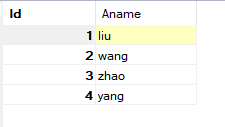``````select * from z_Btable
``````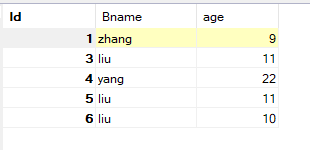A和B的內连接，即笛卡尔积：

``````select * from z_Atable join z_Btable
``````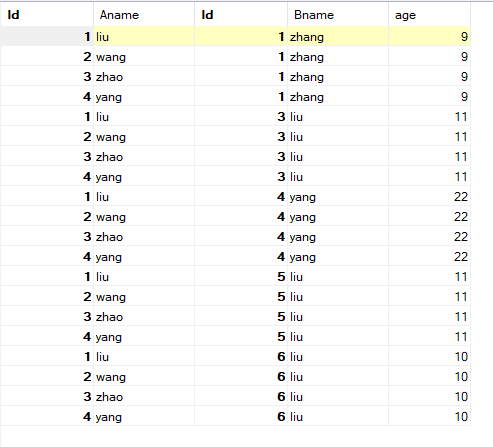``````select * from z_Atable left join z_Btable on 0
``````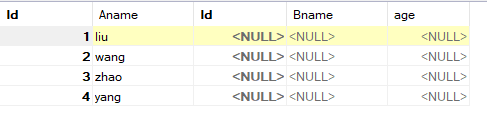``````select * from z_Atable a left join z_Btable b on a.Aname = b.Bname
``````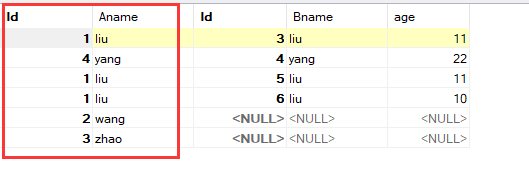``````select * from z_Btable a left join z_Atable b on a.Bname = b.Aname
``````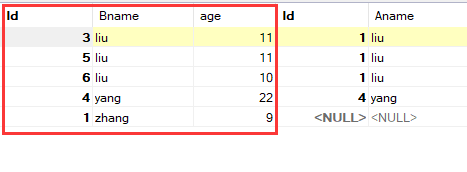;